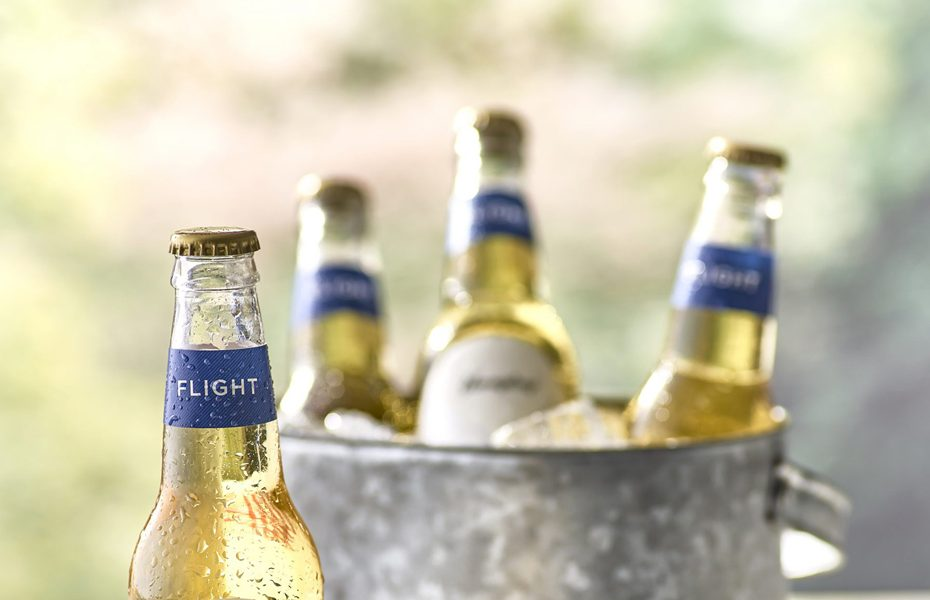# Calories Light BeerCalorie Content Chart Showing Caloric Values for Light Beer

## Calorie Table for Light Beer

Please note: all calorie values are approximate

## Quick Estimate of Caloric Needs For Adult Men

• If moderately active, multiply your current weight in pounds by 16.5
• Or, multiply your weight in kilos by 24, then multiply by 1.5.

## So at 180 pounds your energy needs are about 2970 calories/day

Note: This is a very rough estimate.

• If typically inactive, multiply your current weight in pounds by 14
• Or, multiply your current weight in kilos by 24, then multiply by 1.3.

## So at 200 pounds your energy needs are about 2800 calories/day

Note: This is a very rough estimate.